The Learning Point‎ > ‎Mathematics‎ > ‎

### Applying Vectors to Geometric Problems - Parametric Vectorial equation of a line and Plane, Condition for collinearity of three points, Shortest distance between two lines, Perpendicular distance of a point from a plane or line, Angles between lines and planes#### Introduction to VectorsVectors: Introductory Problems and Examples Applying Vectors to Geometric ProblemsVector Applications in 2D and 3D GeometryVector Differential And Integral Calculus: Theory and DefinitionsVector Differential And Integral Calculus: Solved Problem Sets

------------xxxx------------

Applying Vectors to Geometric Problems

## Vectors and Geometry

### Parametric Vectorial equation of a line

Parametric Vectorial equation of a lineabra b

### Parametric Vectorial equation of a line

Parametric Vectorial equation of a line a br a(b-a)

### Condition for collinearity of three points

Condition for collinearity of three points : The necessary and sufficient condition for three points A, B, C with position vectors a, b, c,x, y, z,xa + yb + zc = 0, x + y + z = 0

### Parametric Vectorial equation of a plane

Parametric Vectorial equation of a plane which passes through the point with position vector abcr ab
a & b cr = a + (b-a) +c

We will also discuss :

### Condition for coplanarity of four points

Condition for coplanarity of four points : The necessary and
sufficient condition for four points A, B, C, D with position vectors a,
b, c, dabcd

### Normal form of vector equation of a plane

The equation r.n
normal to the vector, n.  q
Equation of a plane which is normal to the vector, n, and passing through a point A, with position vector a is (r-a).n

### Plane co-axal with given planes

Equation of a plane passing through the line of intersection of two given planes r.n1 r.n2
(r.n1 – q1). (a.n2– q2) = (r.n2– q2). (a.n1 – q1)

### Angle between two lines

Angle between two lines : Let l1, m1, n1; l2, m2, n2 be the direction cosines of two given lines. The vectors of unit length along given (l1)ijkijk
cosθ = l1 l2+ m1 m2+ n1 n2

### Angle between two planes

Angle between a line and a plane.
r.n1, r.n2 is

θ = cos-1 (n1).(n2)|n1||n2|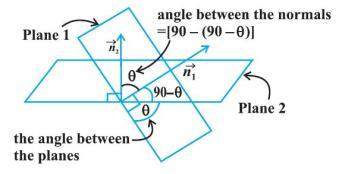### Equation of a plane

which passes through the point with position vector a, and which is parallel to the vectors b and c is also given by [r b c] = [a b c]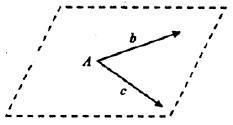r.[bxc + cxa] = [a b c]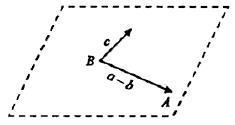(r-a)x[(b-a)x(c-a)] = 0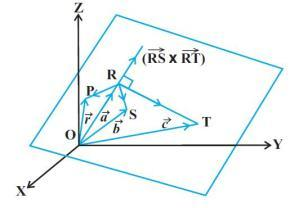### Coplanarity of two lines :

r = a + tb, r = c + pd are coplanar if

[c  b  d] = [a  b  d]

### Shortest distance between two lines :

r = a + tb, r = c + pd is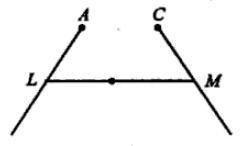LM = |(c-a).(bxd)||bxd| = |[c b d]- [a b d]||bxd|

### Normal form of Cartesian equation of a plane (r.n = q), where r=xi+yj+zk, n = ai + bj + ck is ax + by + cz = p or ax + by + cz + d = 0

#### We will also discuss how to find : Equation of a plane passing through three points P1(x1, y1, z1), P2(x2, y2, z2), P3(x3, y3, z3), Intercept form of equation of a planewhich makes intercepts a, b, c on x, y, z axes, Equation of a line which passes through a point A(x1, y1, z1) having direction ratios p, q, r, Equation of a line which passes through two points P1(x1, y1, z1) and P2(x2, y2, z2), Coplanarity of two lines .

Perpendicular distance of a point (A, with position vector a) from a plane

In case you're interested in learning more about Vectors, here's the full set of tutorials we have :

 Vectors 1a ( Theory and Definitions: Introduction to Vectors; Vector, Scalar and Triple Products) Vectors 1b ( Solved Problem Sets: Introduction to Vectors; Vector, Scalar and Triple Products ) Vectors 2a ( Theory and Definitions: Vectors and Geometry ) Vectors and geometry. Parametric vectorial equations of lines and planes. Angles between lines and planes. Co-planar and collinear points. Cartesian equations for lines and planes in 3D. Vectors 2b ( Solved Problem Sets: Vectors and Geometry ) Vectors 3a ( Theory and Definitions: Vector Differential and Integral Calculus ) Vectors 3b ( Solved Problem Sets: Vector Differential and Integral Calculus )